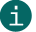# Check digit calculation of Modulo 11

Modulo 11

## This is a description of calculating check digits according to Modulo 11.ActiveBarcode calculates this check digits for most codes automatically.
This documentation is only for the sake of completeness.

Calculation of a checksum according to Modulo 11:
A check digit according to Modulo 11 is used e.g. by PZN. A Modulo 11 is also used for ISBN.

PZN
First all digits are multiplied individually with a multiplier. The multiplier corresponds to the position of the digit + 1. All resulting products are added. The result is then divided by 11. The resulting remainder is the check digit. If the check digit is 10, this number shall not be used as PZN. The code would therefore be invalid.

A calculation example:

 Digits: 6 3 1 9 4 2 Weight: 2 3 4 5 6 7 Results: 12+9+4+45+24+14 = 108 Calculate checksum: 108 / 11 = 9 Remainder 9 Check digit 9

The ISBN-10 and the ISSN checksum
First all digits are multiplied individually with a multiplier. The multiplier corresponds to the position of the digit + 1 from the right. All resulting products are added. The result is then divided by 11. The resulting remainder is subtracted from 11 and results in the check digit.
If result 11 is calculated for this checksum procedure, the checksum is 0. As result the 0 could not be calculated, because in the previous step (remainder with division by 11) 11 is impossible as a result.
It may also happen that the digit 10 is required. 10 is represented by an X: "3-928444-00-X".

A calculation example:

 Digits: 3 9 2 8 4 4 4 0 4 Weight: 10 9 8 7 6 5 4 3 2 Results: 30 + 81 + 16 + 56 + 24 + 20 + 16 + 0 + 8 = 251 Calculate checksum: 251 / 11 = 22 Remainder 9 -> 11 - 9 = 2 Check digit 2